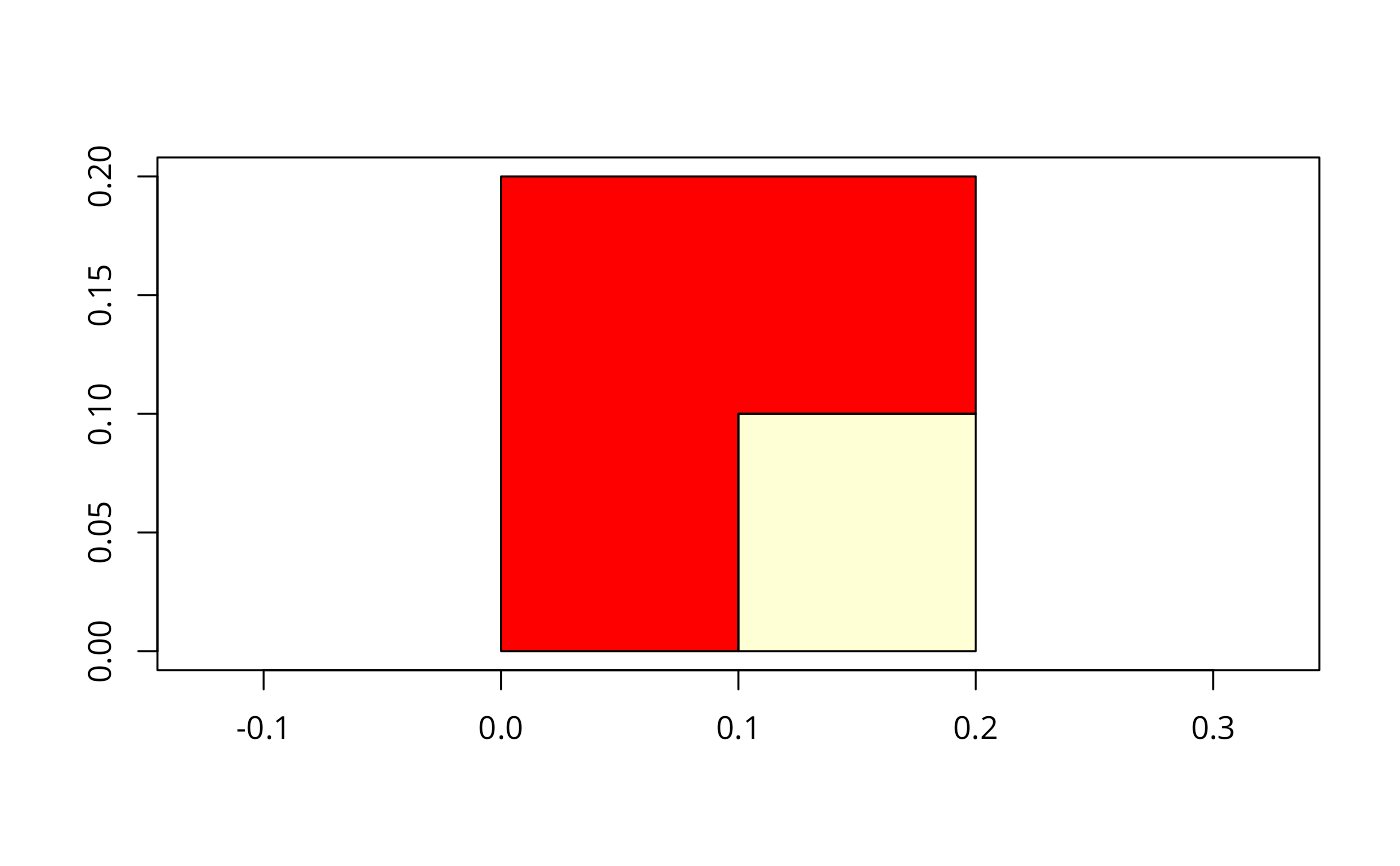Functions for joining intersecting geometries.

gUnionCascaded(spgeom, id = NULL)
gUnaryUnion(spgeom, id = NULL, checkValidity=NULL)
gLineMerge(spgeom, byid=FALSE, id = NULL)

## Arguments

byid

Logical vector determining if the function should be applied across ids (TRUE) or the entire object (FALSE) for spgeom1 and spgeom2

id

Character vector defining id labels for the resulting geometries, if unspecified returned geometries will be labeled based on their parent geometries' labels; it may contain NA values for input objects not included in the union; it should define the memberships of the output Polygons objects

spgeom

sp Polygon(s) or Line(s) depending on the function used

checkValidity

default NULL, integer 0L (no action), 1L (check), 2L (check and try to buffer by zero distance to repair). If NULL, a value set to 0L for GEOS < 3.7.2, 1L for GEOS >= 3.7.2 is read from values assigned on load. Error meesages from GEOS do not say clearly which object fails if a topology exception is encountered. If this argument is > 0L, gIsValid is run on each in turn

## Details

gUnionCascaded expects a single sp object of class SpatialPolygons with subgeometries which it unions together. gUnionCascaded can only dissolve MultiPolygon objects, so GeometryCollection objects to be dissolved, here a SpatialPolygons object, must be flattened a Polygons object; if GEOS version 3.3.0 is available, use gUnaryUnion.

gUnaryUnion expects a single sp object of class SpatialPolygons with subgeometries which it unions together; introduced in GEOS version 3.3.0, and handles GeometryCollection objects. If the id argument is used, it should be a character vector defining the memberships of the output Polygons objects, equal in length to the length of the polygons slot of spgeom.

gLineMerge is similar to gUnionCascaded but is written to work with lines, specifically it joins line segments with intersecting end points.

## Note

Error messages from GEOS, in particular topology exceptions, report 0-based object order, so geom 0 is spgeom1, and geom 1 is spgeom2.

## Author

Roger Bivand & Colin Rundel

gDifference gIntersection gSymdifference

## Examples

if (require(maptools)) {
lps <- coordinates(nc1)
ID <- cut(lps[,1], quantile(lps[,1]), include.lowest=TRUE)
if (version_GEOS0() < "3.3.0") {
} else {
reg4 <- gUnaryUnion(nc1, ID)
}
row.names(reg4)
par(mfrow=c(2,1))
plot(nc1)
plot(reg4)
par(mfrow=c(1,1))
}
#> Warning: shapelib support is provided by GDAL through the sf and terra packages among othersgt <- GridTopology(c(0.05,0.05), c(0.1,0.1), c(2,2))
set.seed(1)
xv <- rnorm(length(coordinates(gt)[,1]))
xvs <- ifelse(xv > 0.2,1,0)
grd <- SpatialGridDataFrame(gt, data.frame(xvs))
spix <- as(grd, "SpatialPixelsDataFrame")
spol <- as(spix, "SpatialPolygonsDataFrame")
image(grd, axes=TRUE)
if (version_GEOS0() < "3.3.0") {
spol1 <- gUnionCascaded(spol, as.character(spol$xvs)) } else { spol1 <- gUnaryUnion(spol, as.character(spol$xvs))
}